# Combinatorics 2012-13

Organisers:

No next seminar

### Term 3 2012/13 - Room MS.04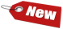Date Name Title
Apr 26, 2013 No seminar (organisers away)
May 3, 2013 Diane Maclagan (Warwick) Combinatorics of the moduli space of phylogenetic trees
May 10, 2013 Florian Lehner (TU Graz) Symmetry breaking in graphs
May 17, 2013 Roman Glebov (Warwick) Conflict-free coloring of graphs
May 24, 2013 Armindo Costa (Warwick) The topology of random uniform hypergraphs
May 31, 2013 No seminar (speaker cancelled)
Jun 7, 2013 Łukasz Grabowski (Oxford) Combinatorics related to spectral theory of random walks on lamplighter groups
Jun 14, 2013 Carl Yerger (Davidson) Steinberg's Conjecture, the Bordeaux Coloring Conjecture and Near-Coloring
Jun 21, 2013 Juraj Stacho (Warwick) Obstructions to intersection families of graphs
Jun 28, 2013 No seminar (organisers away)

### Term 2 2012/13 - Room B1.01

Date Name Title
Jan 11, 2013 Tereza Klimošová (Warwick) Hereditary properties of permutations are strongly testable
Jan 18, 2013 Endre Csóka (Budapest) Local algorithms on bounded degree graphs
Jan 25, 2013 No seminar (room conflict)
Feb 1, 2013 Andrzej Grzesik (Krakow) Flag algebra calculus
Feb 8, 2013 Teresa Sousa (Lisbon) Monochromatic Kr-decompositions of graphs
Feb 15, 2013 John Haslegrave (Sheffield) Judicious partitions of uniform hypergraphs
Feb 22, 2013 Vadim Lozin (Warwick) Ramsey Theory and Parameterized Complexity
Mar 1, 2013 Jan Volec (Warwick) A problem of Erdős and Sós on 3-graphs
Mar 8, 2013 Roman Kotecký (Warwick) Random graph homomorphisms and phase transitions
Mar 15, 2013 Peter Allen (LSE) Embedding and counting in sparse graphs

### Term 1 2012/13 - Room MS.03

Date Name Title
Oct 5, 2012 Daniel Kráľ (Warwick) Quasirandom permutations
Oct 12, 2012 Bruce Westbury (Warwick) Circle Packing
Oct 19, 2012 Agelos Georgakopoulos (Warwick) Random walks on graphs, and the Kirchhoff and Wiener Index
Oct 26, 2012 Jan Hladký (Warwick) f-vectors of three-dimensional flag Gorenstein* complexes via extremal graph theory
Nov 2, 2012 Felipe Rincón (Warwick) Tropical linear spaces, matroid polytopes, and applications
Nov 9, 2012 Matthew Yancey (UIUC) Ore's conjecture on color-critical graphs is almost true
Nov 16, 2012 Daniel Ueltschi (Warwick) Generating functions of connected and 2-connected graphs, and the virial expansion of statistical mechanics
Nov 23, 2012 Oleg Verbitsky (Berlin) Logical complexity of graphs (slides available)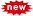Nov 30, 2012 Matthias Lenz (Oxford) Interpolation, box splines, and lattice points in zonotopes
Dec 7, 2012 Stephen Tate (Warwick) Combinatorial Species of Structure and Cluster Expansions

## AbstractsDate: Oct 5, 2012

Title: Quasirandom permutations

Speaker: Daniel Kráľ (Warwick)

A systematic study of large combinatorial objects has recently led to discovering many connections between discrete mathematics and analysis. In this talk, we explore the analytic view of large permutations. We associate every sequence of permutations with a measure on a unit square and show the following: if the density of every 4-element subpermutations in a permutation p is 1/4!+o(1), then the density of every k-element subpermutation is 1/k!+o(1). This solves a question of Graham whether quasirandomness of a permutation is captured by densities of its 4-element subpermutations. The talk is based on joint work with Oleg Pikhurko.Date: Oct 12, 2012

Title: Circle Packing

Speaker: Bruce Westbury (Warwick)

I will present the circle packing theorem (also known as the Koebe-Andreev-Thurston theorem) and its applications to the Riemann mapping theorem and to Belyi theory. This theorem has a wiki page http://en.wikipedia.org/wiki/Circle_packing_theorem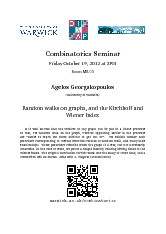Date: Oct 19, 2012

Speaker: Agelos Georgakopoulos (Warwick)

It is well known that the vertices of any graph can be put in a linear preorder so that, for random walk on the graph, vertices appearing earlier in the preorder are “easier to reach but more difficult to get out of”. We exhibit further such preorders corresponding to various functions related to random walk, and study their relationships. These preorders coincide when the graph is a tree, but not necessarily otherwise. In the case of trees, we prove a simple identity relating hitting times to the Wiener index. The original motivation for this work was the study of cover time, and a connection will be shown. Joint with S. Wagner (Stellenbosch)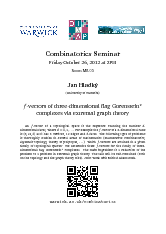Date: Oct 26, 2012

An f-vector of a topological space is the sequence counting the number d-dimensional faces, where d=0,1,.... For example the f-vector of a 3-dimensional cube is (8,12,6) as it has 8 vertices, 12 edges and 6 faces. The following type of problems is thoroughly studied in several areas of mathematics (enumerative combinatorics, algebraic topology, theory of polytopes, ...): which f-vectors are attained in a given family of topological spaces? We determine these f-vectors for the family of three-dimensional flag Gorenstein* complexes. The main ingredient is a reduction of the problem to a problem in extremal graph theory. The talk will be self-contained (both on the topology and the graph theory side). Joint work with Michal Adamaszek (arXiv:1205.4060).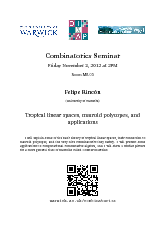Date: Nov 2, 2012

Speaker: Felipe Rincón (Warwick)

I will explain some of the basic theory of tropical linear spaces, their connection to matroid polytopes, and the very nice combinatorics they satisfy. I will present some applications to computational commutative algebra, and I will show a similar picture for a more general class of matroids called Coxeter matroids.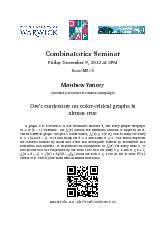Date: Nov 9, 2012

Speaker: Matthew Yancey (UIUC)

A graph G is k-critical if it has chromatic number k, but every proper subgraph of G is (k – 1)-colorable. Let fk(n) denote the minimum number of edges in an n-vertex k-critical graph. We give a lower bound fk(n) ≥ F(k,n) that is sharp for every n = 1 mod (k – 1). It is also sharp for k = 4 and every n ≥ 6. The result improves the classical bounds by Gallai and Dirac and subsequent bounds by Krivelevich and Kostochka and Stiebitz. It establishes the asymptotics of fk(n) for every fixed k. It also proves that the conjecture by Ore from 1967 that for every k ≥ 4 and nk + 2, fk(n + k – 1) = fk(n) +
k – 1
____
2
(
k
2
____
k – 1
)
holds for each k ≥ 4 for all but at most k3/12 values of n. This is joint work with Alexandr Kostochka.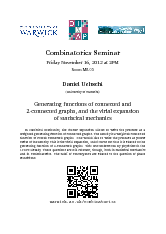Date: Nov 16, 2012

Speaker: Daniel Ueltschi (Warwick)

In statistical mechanics, the cluster expansion allows to write the pressure as a weighted generating function of connected graphs. The density is a weighted connected function of rooted connected graphs. One would like to write the pressure as power series of the density. This is the virial expansion, and it turns out that it is related to the generating function of 2-connected graphs. This was understood by physicists in the 1930's already. These questions are still relevant, though, both in statistical mechanics and in combinatorics. The radii of convergence are related to the question of phase transitions.Date: Nov 23, 2012

Speaker: Oleg Verbitsky (Berlin)

We discuss the definability of finite graphs in first-order logic with two relation symbols for adjacency and equality of vertices. The logical depth D(G) of a graph G is equal to the minimum quantifier depth of a sentence defining G up to isomorphism. The logical width W(G) is the minimum number of variables occurring in such a sentence. The logical length L(G) is the length of a shortest defining sentence. We survey known estimates for these graph parameters and discuss their relations to other topics, with emphasis on the Weisfeiler-Lehman algorithm in isomorphism testing.

Download: slides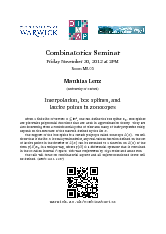Date: Nov 30, 2012

Speaker: Matthias Lenz (Oxford)

Given a finite list of vectors X⊆ℝd, one can define the box spline BX. Box splines are piecewise polynomial functions that are used in approximation theory. They are also interesting from a combinatorial point of view and many of their properties solely depend on the structure of the matroid defined by the list X. The support of the box spline is a certain polytope called zonotope Z(X). We will show that if the list X is totally unimodular, any real-valued function defined on the set of lattice points in the interior of Z(X) can be extended to a function on Z(X) of the form p(D)BX in a unique way, where p(D) is a differential operator that is contained in the so-called internal P-space. This was conjectured by Olga Holtz and Amos Ron. The talk will focus on combinatorial aspects and all objects mentioned above will be defined. (arXiv:1211.1187)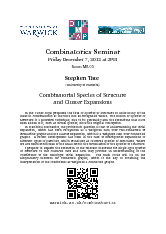Date: Dec 7, 2012

Speaker: Stephen Tate (Warwick)

In the 1980s Joyal proposed the idea of Species of Structure to unite many of the ideas in combinatorics at the time into an integrated whole. The notion of Species of Structure is a powerful technique, due to its generality and the extensions that have been added to it, such as virtual species, since the original conception. In Statistical Mechanics, the problem in question is that of understanding the Virial Expansion, which has been recognised as a weighted sum over two-connected or irreducible graphs and the Cluster Expansion, which is a weighted sum over connected graphs. A recent development has been in the case of multispecies expansions of different types of particles, which translates as coloured species of structures, where we use multisets instead of the usual sets in the formulation of the Species of Structure. I propose to explain the extension of the relevant theorems for single-type species of structure to this coloured case and how they provide an understanding of the coefficients of the multitype Virial Expansion. The main focus will be on the dissymmetry theorem for connected graphs, which is the key to obtaining the interpretation of the coefficients as weighted 2-connected graphs.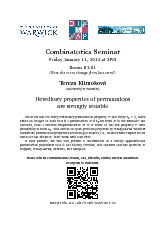Date: Jan 11, 2013

Speaker: Tereza Klimošová (Warwick)

We show that for every hereditary permutation property P and every ε > 0, there exists an integer M such that if a permutation π is ε-far from P in the Kendall's tau distance, then a random subpermutation of π of order M has the property P with probability at most ε. This settles an open problem proposed by Kohayakawa whether hereditary permutation properties are strongly testable, i.e., testable with respect to the Kendall's tau distance. Joint work with Dan Kráľ. If time permits, we will also present a construction of a finitely approximable permutation parameter that is not finitely forcible; this answers another question of Hoppen, Kohayakawa, Moreira, and Sampaio.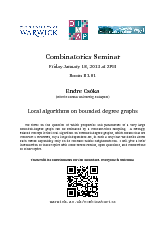Date: Jan 18, 2013

Speaker: Endre Csóka (Budapest)

We focus on the question of which properties and parameters of a very large bounded-degree graph can be estimated by a constant-time sampling. A strongly related concept is the local algorithm on bounded-degree graphs, which means that we construct a structure, say a large independent set, in such a way that we decide about each vertex depending only on its constant radius neighbourhood. I will give a brief introduction to these topics with some recent results, open questions, and connections to other topics.Date: Feb 1, 2013

Title: Flag algebra calculus

Speaker: Andrzej Grzesik (Krakow)

During the talk I will present basic definitions and intuitions related to flag algebras, which gives a very powerful tool in Extremal Combinatorics. I will also prove some theorems using this technique.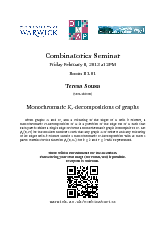Date: Feb 8, 2013

Speaker: Teresa Sousa (Lisbon)

Given graphs G and H, and a colouring of the edges of G with k colours, a monochromatic H-decomposition of G is a partition of the edge set of G such that each part is either a single edge or forms a monochromatic graph isomorphic to H. Let φk(n,H) be the smallest number t such that any graph G of order n and any colouring of its edges with k colours admits a monochromatic H-decomposition with at most t parts. Results for the function φk(n,Kr) for k≥2 and r≥3 will be presented.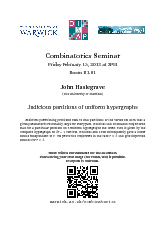Date: Feb 15, 2013

Speaker: John Haslegrave (Sheffield)

Judicious partitioning problems seek to find partitions of the vertex set such that a given parameter is reasonably large for every part. Bollobás and Thomason conjectured that for a particular problem on r-uniform hypergraphs the worst case is given by the complete hypergraph on 2r – 1 vertices. Bollobás and Scott subsequently gave a lower bound independent of r. We prove the conjecture in the case r = 3 and give improved bounds for r > 3.Date: Feb 22, 2013

Parameterized complexity is a recent branch of computational complexity theory that provides a framework for a refined analysis of hard algorithmic problems. Ramsey theory is a branch of mathematics that studies the conditions under which order must appear. In this talk, we will reveal various connections between the two fields.Date: Mar 1, 2013

Speaker: Jan Volec (Warwick)

We show that for every ε > 0 there exist δ > 0 and n0 ∈ ℕ such that every 3-uniform hypergraph on nn0 vertices with the property that every k-vertex subset, where k ≥ δn, induces at least (1/4 + ε) (k 3) edges, contains K4 as a subgraph, where K4 is the 3-uniform hypergraph on 4 vertices with 3 edges. This question was originally raised by Erdős and Sós. The constant 1/4 is the best possible. Joint work with Roman Glebov and Daniel Kráľ.Date: Mar 8, 2013

Speaker: Roman Kotecký (Warwick)

The main idea of phase transition stemming from entropic long range order will be explained and illustrated in the case of random colourings on a class of planar quasi-transitive graphs. Based on joint works with A. Sokal and J. Swart.Date: Mar 15, 2013

Speaker: Peter Allen (LSE)

There has been substantial interest in the last fifteen years in extremal graph theory `relative to' sparse random or pseudorandom graphs: for instance, what fraction of the edges of the random graph Gn,p must we delete in order to remove all triangles? This question is just Turán's theorem in the `dense case' p=1, but is non-trivial when p << 1. Following the work of Conlon and Gowers, and Schacht, we can give a detailed answer to the above question not only for triangles but for any fixed graph H; more generally, we now (due to Conlon, Gowers, Samotij and Schacht) have a `Sparse Counting Lemma' for random graphs complementing the existing `Sparse Regularity Lemma' of Kohayakawa and independently Rödl. For pseudorandom graphs, however, we know much less. I will describe the current best `Sparse Counting Lemma' for pseudorandom graphs, improving recent work of Conlon, Fox and Zhao (joint work with Julia Boettcher, Jozef Skokan and Maya Stein). Classical extremal graph theory also deals with `Dirac-type' problems where one asks for conditions on G to contain some large, or spanning, subgraph H (such as a Hamilton cycle). For these problems it is typically necessary to invoke the Szemerédi Regularity Lemma together with the Blow-up Lemma. In order to solve the corresponding problems relative to sparse graphs, one therefore requires a sparse version of the latter. The natural generalisation of the Blow-up Lemma to sparse graphs is however false. I will explain what a Blow-up Lemma is, why it fails for sparse graphs, and what we can prove (joint with Julia Boettcher, Hiep Han, Yoshiharu Kohayakawa and Yury Person).Date: May 3, 2013

Speaker: Diane Maclagan (Warwick)

A phylogenetic tree is a tree with labelled leaves. The space of phylogenetic trees is a simplicial complex (or more generally a simplicial fan) that records the lengths of internal edges of the trees. This arose from mathematical biology but has applications in a range of areas. In this talk, after introducing this space and describing its combinatorial structure, I will focus on some geometric combinatorial questions about it that have applications in algebraic geometry to the geometry of moduli spaces of genus zero curves. Algebraic geometry will only enter at the end of the talk, and no background will be assumed.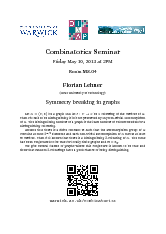Date: May 10, 2013

Speaker: Florian Lehner (TU Graz)

Let G = (V, E) be a graph and let c: VC be a colouring of the vertices of G. Then c is said to be distinguishing if it is not preserved by any non-trivial automorphism of G. The distinguishing number of a graph is the least number of colours needed for a distinguishing colouring. Assume that there is a finite constant m such that the automorphism group of G contains at most 2m/2 elements and each non-trivial automorphism of G moves at least m vertices. Then it is known that there is a distinguishing 2-colouring of G. The same has been conjectured to be true for locally finite graphs and m = ℵ0. We give several classes of graphs where this conjecture is known to be true and show that random 2-colourings have a good chance of being distinguishing.Date: May 17, 2013

Speaker: Roman Glebov (Warwick)

When talking about coloring a graph, the by far most studied notion is the proper coloring, that is, a coloring of the vertex set such that the color of each vertex is unique in the vertex's closed neighborhood. We study a generalization of this concept, calling a coloring conflict-free if the closed neighborhood of each vertex contains a vertex with a color that is unique there. We study the conflict-free chromatic number χCF of graphs from the extremal and probabilistic points of view. We resolve a question of Pach and Tardos about the maximum conflict-free chromatic number an n-vertex graph can have. Our construction is randomized. In relation to this we study the evolution of the conflict-free chromatic number of the Erdős-Rényi random graph G(n,p) and give the asymptotics for p = ω(1/n). We also show that for p ≥ 1/2 the conflict-free chromatic number typically differs from the domination number by at most 3. Joint work with Tibor Szabó and Gábor Tardos.Date: May 24, 2013

Speaker: Armindo Costa (Warwick)

In this talk I will describe some models for random simplicial complexes introduced in recent literature. These can be viewed as random k-uniform hypergraphs. Although these models generalize the celebrated Erdős-Rényi model for random graphs, their higher dimension motivates the study of probabilistic topology, i.e. estimating topological invariants of random spaces. I will survey some of the known topological phase transitions, connections to Gromov's theory of random groups and some challenges that lay ahead.Date: Jun 7, 2013

Speaker: Łukasz Grabowski (Oxford)

Recently there was a considerable amount of interest in computing spectral properties of random walk operators (and more generally - group ring operators) on Cayley graphs of lamplighter-type groups. In all cases such computations require some non-trivial input from combinatorics. For example, Lehner and Wagner computed the kernel dimension of a random walk operator on the free lamplighter group by being able to explicitly write down the generating function for the size of the maximal matching in finite trees. In the talk I will explain how the relation between the spectrum of the random walk and combinatorics is usually established. After briefly surveying some of the success stories of this technique I'll focus on presenting two elementary combinatorial problems whose solutions would give some new information about random walk operators. One is related to the generating functions of languages in the complexity class LOGSPACE, and the other is related to coefficients of noncommutative rational functions whose coefficients are powers of a fixed prime number.Date: Jun 14, 2013

Speaker: Carl Yerger (Davidson)

An important result in the theory of graph coloring is Grotzsch's theorem, which states that every triangle-free planar graph is 3-colorable. A famous related question is due to Steinberg and states that any planar graph without 4- or 5-cycles is 3-colorable. There has been considerable recent interest in trying to prove this conjecture. In this talk, we will discuss some of the recent progress made towards proving Steinberg’s conjecture and discuss recent joint work with Ken-ichi Kawarabayashi that planar graphs with no 5-cycles, 6-cycles or intersecting triangles are 3-colorable. In addition, we discuss related senior thesis based on near-coloring with Davidson student Kyle Yang.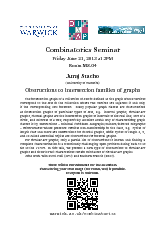Date: Jun 21, 2013

Speaker: Juraj Stacho (Warwick)

The intersection graph of a collection of sets is defined as the graph whose vertices correspond to the sets in the collection where two vertices are adjacent if and only if the corresponding sets intersect. Many popular graph classes are characterised as intersection graphs of particular types of sets, e.g. interval graphs, circular-arc graphs, chordal graphs are the intersection graphs of intervals of the real line, arcs of a circle, and subtrees of a tree, respectively. Another useful way of characterising graph classes is by obstructions—minimal forbidden subgraphs/minors/induced subgraphs—substructures whose presence certifies non-membership in the class, e.g. cycles of length four and more are obstructions for chordal graphs, while cycles of length 4, 5, and so-called asteroidal triples are obstructions for interval graphs. For circular-arc graphs, only a partial list of obstructions is known and finding a complete characterisation is a notoriously challenging open problem dating back to as far as the 1970's. In this talk, we present a new type of obstructions to circular-arc graphs and show how it characterises certain subclasses of circular-arc graphs. Joint work with Pavol Hell (SFU) and Mathew Francis (IMSc).